Chapter Notes: Introduction to Euclid`s Geometry

# Introduction to Euclid`s Geometry Chapter Notes - Mathematics (Maths) Class 9

## Introduction

The word ‘geometry’ comes from the Greek word ‘geo’, meaning the ‘earth’, and ‘metron’, meaning ‘to measure’. Thus, the word ‘geometry’ means ‘earth measurement’.

• Ancient Egyptians were known to be the first people to study geometry.
• They developed several geometric techniques and rules for calculating simple areas and also for doing simple constructions.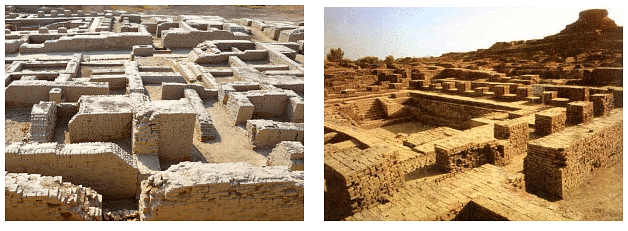• In the Indian subcontinent, the mining at Harappa and Mohenjo-Daro, etc. show that the Indus Valley Civilization (about 3000 BC) made extensive use of geometry. It was a highly organized society. These cities were highly developed and very well planned.
• Therefore, geometry was being developed and applied everywhere in the world.

A Greek mathematician, Thales is credited with giving the first known proof that a circle is cut into two equal parts by its diameter.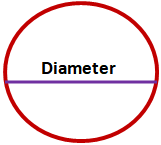• Around 300 BC, Euclid a teacher of mathematics at Alexandria in Egypt, collected all the known work and arranged it in his famous treatise, called ‘Elements’. He divided the ‘Elements’ into thirteen chapters, each called a book.

### Euclid’s Definitions, Axioms

During Euclid’s time, the mathematicians got the idea of the point, line, plane (or surface) from the study of space and solids in the space around them. Thus, an abstract geometrical notion of a solid object was developed.

• A solid has shape, size, and position and can be moved from one place to another.
• Its boundaries are called surfaces.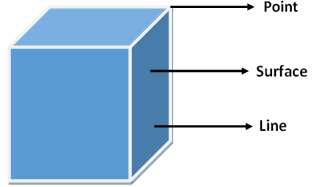• They separate one part of the space from another and are said to have no thickness.
• The boundaries of the surfaces are curved or straight lines. These lines end in points.Some of the other assumptions or definitions listed by Euclid’s are:
(i) A point is that which has no part.
(ii) A line is a breadthless length.
(iii) The ends of a line are points.
(iv) A straight line is a line which lies evenly with the points on itself.
(v) A surface is that which has length and breadth only.
(vi) The edges of a surface are lines.
(vii) A plane surface is a surface which lies evenly with the straight lines on itself.
Now, consider the first definition of a point. In this definition, a part needs to be defined. If we define ‘part’ to be something which occupies ‘area’, then again, we have to define the area. So, if we define these terms, we get a chain of definitions without an end. Therefore, the mathematicians agreed to leave some geometric terms undefined.
Similarly, the second definition of a line refers to breadth and length which have not been defined.
So, in geometry, we take a point, a line and a plane (or a plane surface) as undefined terms.
Starting with his definitions, Euclid assumed certain properties, which were not to be proved. These assumptions are actually ‘obvious universal truths’.
He divided them into two types: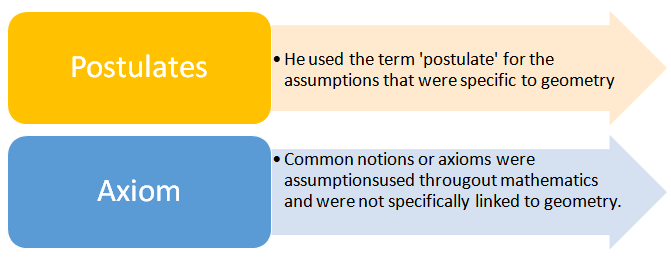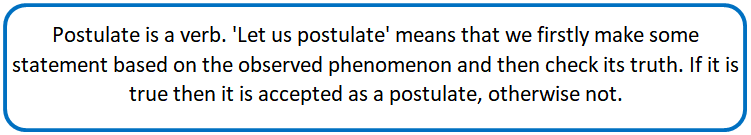Some of Euclid’s axioms are:
(1) Things which are equal to the same thing are equal.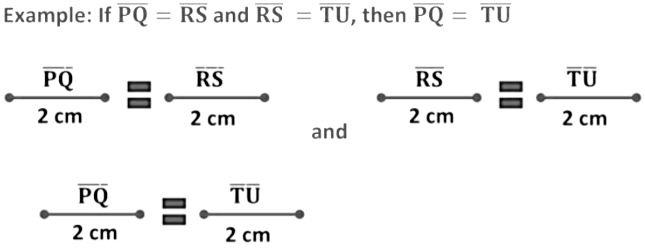So,
If line segment PQ is equal to line segment RS and line segment RS is equal to line segment TU, then line segment PQ is equal to line segment TU.

(2) If equals are added to equals, the wholes are equal.
Two jars, A and B have the same quantity of sugar, which is 2 kg.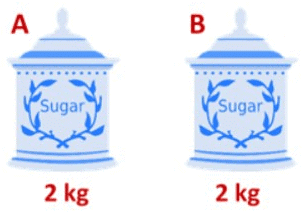Now, we add 1 kg of sugar to both the jars, A and B.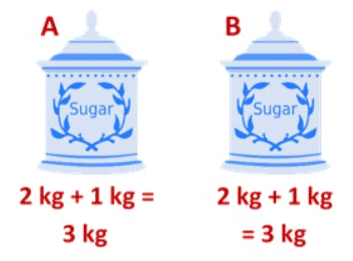We see that the final quantity of sugar is the same in both the jars.

(3) If equals are subtracted from equals, the remainders are equal.
We again take the example of two jars having the same quantity of sugar (2 kg).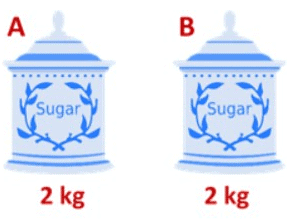In this case, we remove 1 kg of sugar from both the jars, A and B.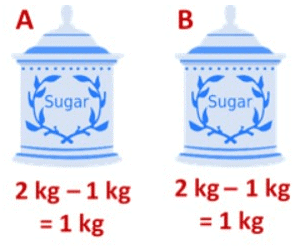We see that the final quantity of sugar in both the jars remains the same.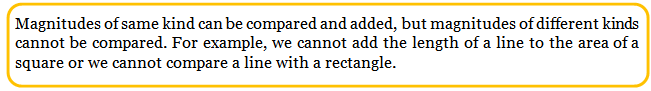(4) Things which coincide with one another are equal.
Two pages of the same book coincide with each other and hence are equal.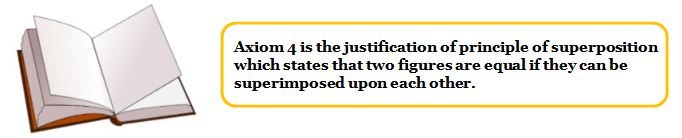(5) The whole is greater than the part.Here, we see that a whole cake is greater than a slice (part) of the cake.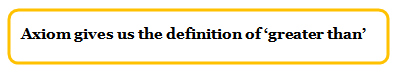(6) Things which are double of the same things are equal.
If x = 2y and z = 2y then x = z .
Suppose there are three jars of sugar, A, B, and C. Jars, A and B have an equal quantity of sugar, that is 2 kg and jar C has 1 kg of sugar.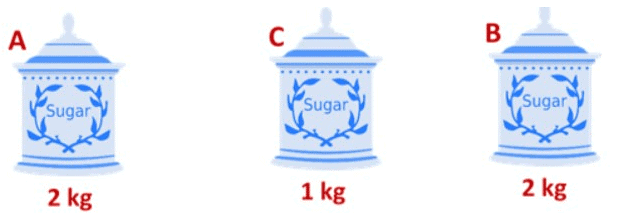So, we see that the quantity of sugar in jar A and B is equal to twice the quantity of sugar in jar C or we can also say that both the jars A and B, have the same quantity of sugar as their quantities are also equal to twice the quantity of sugar in jar C.

(7) Things that are halves of the same things are equal.
If x = y/2 and z = y/2 then x = z.
Suppose there are three jars of sugar, A, B, and C. Jars, A and B have an equal quantity of sugar, that is 1 kg and jar C has 2 kg of sugar.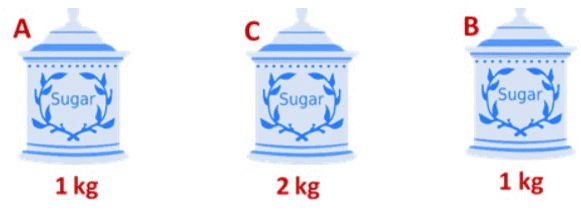So, we see that the quantity of sugar in jar A and B is equal to half the quantity of sugar in jar C or we can also say that both the jars A and B, have the same quantity of sugar as their quantities are also equal to half the quantity of sugar in jar C.

Example 1: Solve the equation y − 10 = 13 and state Euclid’s axioms used here.

y − 10 = 13
y − 10 + 10 = 13 + 10
y = 23
Here we have used Euclid’s axiom 2 which states that if equals are added to equals, the wholes are equals.

Example 2: In the given figure PT = QT, TR = TS, show that PR = QS. Write Euclid’s axiom to support this.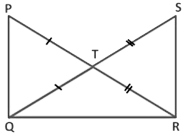It is given that,
PT = QT Eq → 1
TR = TS Eq → 2
To show, PR = QS
Adding Eq 1 and Eq 2 we get,
PT + TR = QT + TS
PR = QS
Here, we have used Euclid’s axiom 2 which states that if equals are added to equals, the wholes are equals.

Example 3: In the given figure, we have BD = BC and AC = BC. State Euclid’s axiom to support this.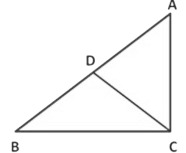If BD = BC and AC = BC, then BD = AC
Here, we have used Euclid’s axiom 1 which states that things which are equal to the same thing are equal.

Example: Eric and David have the same weight. If both of them lose 5 kg weight, how will you compare their new weights?

Let x kg be the initial weight of both Eric and David.
On losing 5 kg, the weight of Eric and David will be (x − 5) kg.
According to Euclid’s axiom 3, if equals are subtracted from equals, the remainders are equal.
So, even after losing 5 kg, both Eric and David will have the same weight as their initial weights are also equal.

### Euclid’s Five Postulates:

Postulate 1: A straight line may be drawn from any one point to any other point

This postulate states that at least one straight line passes through two distinct points, but it does not say that there cannot be more than one such line. However, Euclid has frequently assumed that there is a unique line joining two distinct points.
This result can be stated as an axiom given below:
Axiom: Given two distinct points, there is a unique line that passes through them.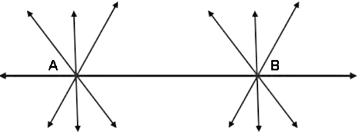Here, we see that only one line, AB is passing through A and also through B. Now can you tell how many lines are passing through B and also through A?
We see that the same line, i.e. AB is passing through B and A. The above statement is self-evident and so, it is called an axiom.

Postulate 2: A terminated line can be produced indefinitely.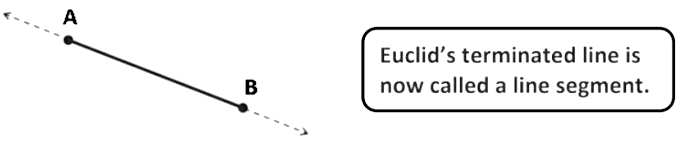Postulate 2: states that a line segment can be extended on either side to form a line.

Postulate 3: A circle can be drawn with any center and any radius.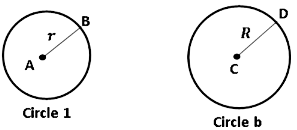Here, AB = r is the radius of circle 1 and CD = R is the radius of circle 2.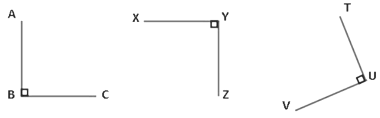Postulate 4: All right angles are equal.
Here, ∠ABC = 90°
∠XYZ = 90°
∠TUV = 90°
So, all the right angles are equal.

Postulate 5: If a straight line falling on two straight lines makes the interior angles on the same side of it taken together less than two right angles (180°), then the two straight lines, if produced indefinitely, meet on that side on which the sum of angles is less than two right angles.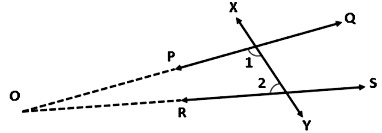Suppose a straight line XY falls on two straight lines, PQ and RS in such a way that the interior angles ∠1 + ∠2 <180° on the left side of XY. The two lines PQ and RS, when produced indefinitely, will eventually intersect at point O on the left side of PQ.
If we see the five postulates, we notice that postulate 5 is more complex than any other postulate. The postulates 1 through 4 are so simple and obvious that these are taken as ‘self - evident truths’. As it is not possible to prove these statements, they are accepted without any proof.
Euclid used his postulates and axioms to prove other results. Then using these results, he proved some more results by applying deductive reasoning. The statements that were proved are called propositions or theorem.
Euclid deduced 465 propositions in a logical chain using his axioms, postulates, definitions, and theorems proved earlier in the chain.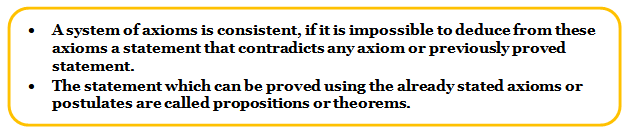Example: If S, T, and U are three points on a line, and T lies between S and U, then prove that ST + TU = SU.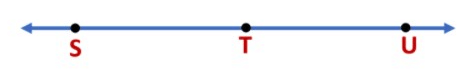We see that SU coincides with ST + TU. According to Euclid’s axiom 4, things that coincide with one another are equal.
Therefore, SU = ST + TU
Now, in this solution, it has been assumed that there is a unique line passing through two points.

Example: Prove that an equilateral triangle can be constructed on any given line segment.
(1) We first draw a line segment PQ of length 1 cm.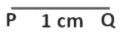(2) Next, we draw a circle with point Q as center and QP as the radius. (Using Euclid’s postulate 3 – A circle can be drawn with any center and any radius)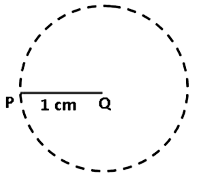(3) Similarly, we draw another circle with P as center and radius equal to PQ.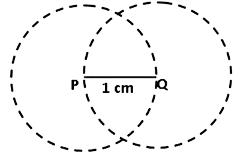(4) The two circles meet at point R. Now draw the line segments, RP and RQ to form the ∆ RPQ.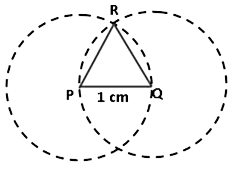To prove:∆ RPQ is an equilateral triangle
Here,
PQ = PR = 1 cm (Radii of the same circle)
PQ = QR = 1 cm (Radii of the same circle)
Using these two facts and Euclid’s axiom 1 (things which are equal to the same thing are equal) we conclude that,
PQ = PR = QR = 1 cm
Therefore, ∆ RPQ is an equilateral triangle as all the sides are equal.
Here, we see that Euclid has assumed that the two circles drawn with centers P and Q will meet each other at a point.

Theorem
Two distinct lines cannot have more than one point in common.
To prove: Lines l and m have only one point in common.
Proof: Let us suppose that the two lines intersect at two distinct points A and B which means that two lines are passing through two distinct points A and B.
According to the axiom, only one line can pass through two distinct points. Therefore, the assumption that the two lines can pass through two distinct points is wrong. So, two distinct lines cannot have more than one point in common.

### Summary of Euclid Geometry

Euclid’s definition
A point is that which has no part.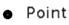A line is a breadthless length. The ends of a line are points and the straight line is a line which lies evenly with the points on itself.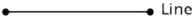A surface is that which has length and breadth only. The edges of a surface are lines.

###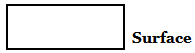Euclid’s axiom
(i) Things which are equal to the same thing are equal.

Example: If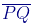=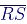and=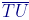, then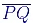=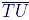(ii) If equals are added to equals, the wholes are equal.

Example: If x = y then x + z = y + z

(iii) If equals are subtracted from equals, the remainders are equal.

Example: If x = y then x − z = y − z

(iv) Things which coincide with one another are equal.

Example: Two pages of the same book

(v) The whole is greater than the part.

Example: A whole cake is greater than a slice (part) of the cake.

(vi) Things which are double of the same things are equal to one another.

Example: If x = 2y and z = 2y then x = z

(vii) Things which are halves of the same things are equal to one another.

Example: If x = y/2 and z = y/2 then x = z.

In geometry, a point, a line and a plane (or a plane surface) are considered as undefined terms.

• Euclid used the term ‘postulate’ for the assumptions that were specific to geometry.
• Common notions or axioms were assumptions used throughout mathematics and were not specifically linked to geometry.

Euclid’s postulate
Postulate 1: A straight line may be drawn from any one point to any other point.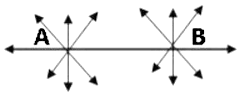Postulate 2: A terminated line can be produced indefinitely.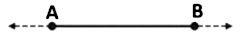Postulate 3: A circle can be drawn with any centre and any radius.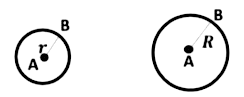Postulate 4: All right angles are equal.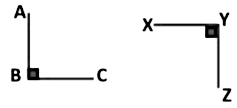Postulate 5: If a straight line falling on two straight lines makes the interior angles on the same side of it taken together less than two right angles (180°), then the two straight lines, if produced indefinitely, meet on that side on which the sum of angles is less than two right angles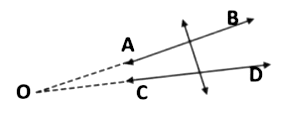Equivalent Versions of Euclid’s Fifth Postulate
‘For every line l and for every point P not lying on l, there exist a unique line m passing through P and parallel to l.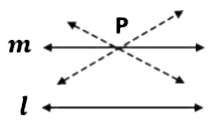The document Introduction to Euclid`s Geometry Chapter Notes | Mathematics (Maths) Class 9 is a part of the Class 9 Course Mathematics (Maths) Class 9.
All you need of Class 9 at this link: Class 9

## FAQs on Introduction to Euclid`s Geometry Chapter Notes - Mathematics (Maths) Class 9

 1. What is Euclid's Geometry?Euclid's Geometry refers to the mathematical system developed by the ancient Greek mathematician Euclid. It is a branch of mathematics that focuses on the study of geometric shapes, lines, angles, and their properties. Euclid's Geometry is based on a set of axioms and postulates that are used to prove various geometric theorems.
 2. What are the main topics covered in Euclid's Geometry?Euclid's Geometry covers a wide range of topics, including points, lines, planes, angles, triangles, quadrilaterals, circles, and their properties. It also includes the study of congruence, similarity, parallel lines, perpendicular lines, and the Pythagorean theorem. Euclid's Geometry is divided into 13 books, each covering different aspects of geometry.
 3. Who was Euclid and why is he important in the field of mathematics?Euclid was a Greek mathematician who lived around 300 BCE. He is often referred to as the "Father of Geometry" due to his significant contributions to the field. Euclid's most famous work is his book, "Elements," which is a comprehensive compilation of mathematical knowledge at that time. His book became the standard textbook for geometry for over 2,000 years and laid the foundation for the study of geometry as we know it today.
 4. What are the axioms and postulates in Euclid's Geometry?The axioms in Euclid's Geometry are basic statements that are accepted as true without proof. They serve as the starting point for all geometric reasoning. The postulates, on the other hand, are specific assumptions or conditions that are used to prove various theorems. Euclid's Geometry is based on five main axioms, including the existence of a straight line between any two points and the ability to extend a line segment indefinitely.
 5. How is Euclid's Geometry relevant in the modern world?Although Euclid's Geometry was developed over 2,000 years ago, its principles and concepts are still widely used in various fields today. It serves as the foundation for many branches of mathematics, including algebra, calculus, and computer graphics. Euclid's Geometry is also applied in architecture, engineering, physics, and computer science. Its emphasis on logical reasoning, proof, and deductive reasoning has shaped the way we approach problem-solving and critical thinking in various disciplines.

## Mathematics (Maths) Class 9

62 videos|426 docs|102 tests

## Mathematics (Maths) Class 9

62 videos|426 docs|102 tests
Signup to see your scores go up within 7 days! Learn & Practice with 1000+ FREE Notes, Videos & Tests.
10M+ students study on EduRev
Track your progress, build streaks, highlight & save important lessons and more!(Scan QR code)
Related Searches

,

,

,

,

,

,

,

,

,

,

,

,

,

,

,

,

,

,

,

,

,

;